# Traingulating Point Clouds

Default UI Menu: Tools/Point Cloud/Point Cloud Triangulation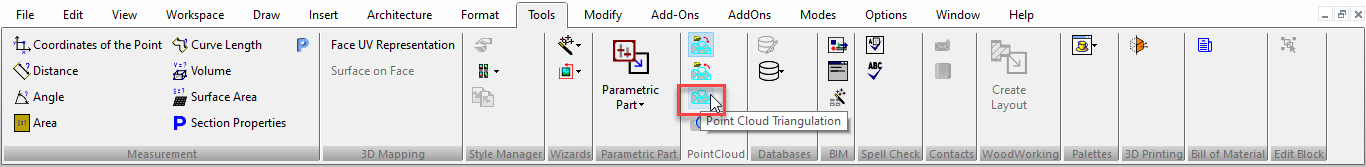This tool is use to create a triangulated mesh from a point cloud. The method works by maintaining a list of points from which the mesh can be grown ("fringe" points) and extending it until all possible points are connected. It can deal with unorganized points, coming from one or multiple scans, and having multiple connected parts. It works best if the surface is locally smooth and there are smooth transitions between areas with different point densities.

1. Select Point Cloud object
2. Define triangulation method parameters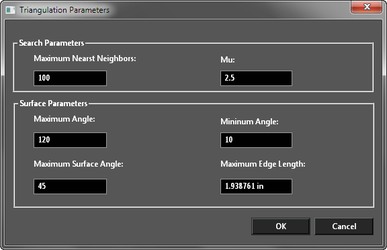1. Click OK.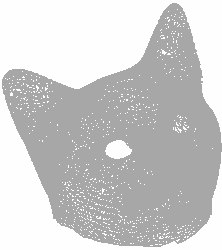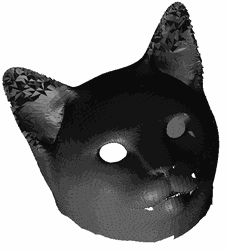The point cloud is retained: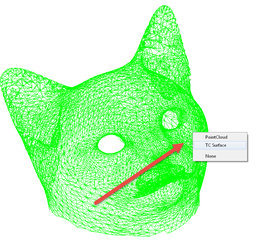Parameters:

Triangulation is performed locally, by projecting the local neighborhood of a point along the point's normal, and connecting unconnected points. Thus, the following parameters can be set:

• Maximum Nearest Neighbors and Mu control the size of the neighborhood. The former defines how many neighbors are searched for, while the latter specifies the maximum acceptable distance for a point to be considered, relative to the distance of the nearest point (in order to adjust to changing densities). Typical values are 50-100 and 2.5-3 (or 1.5 for grids).
• Maximum Edge Length is practically the maximum edge length for every triangle. This has to be set by the user such that to allow for the biggest triangles that should be possible.
• Minimum Angle and Maximum Angle are the minimum and maximum angles in each triangle. While the first is not guaranteed, the second is. Typical values are 10 and 120 degrees (in radians).
• Maximum Surface Angle is meant to deal with the cases where there are sharp edges or corners and where two sides of a surface run very close to each other. To achieve this, points are not connected to the current point if their normals deviate more than the specified angle (note that most surface normal estimation methods produce smooth transitions between normal angles even at sharp edges). This angle is computed as the angle between the lines defined by the normals (disregarding the normal's direction) if the normal-consistency-flag is not set, as not all normal estimation methods can guarantee consistently oriented normals. Typically, 45 degrees (in radians) and false works on most datasets.